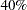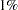The ROBUSTREG Procedure

## Example 74.1 Comparison of Robust Estimates

This example contrasts several of the robust methods available in the ROBUSTREG procedure.

The following statements generate 1000 random observations. The first 900 observations are from a linear model, and the last 100 observations are significantly biased in the-direction. In other words, 10% of the observations are contaminated with outliers.

```   data a (drop=i);
do i=1 to 1000;
x1=rannor(1234);
x2=rannor(1234);
e=rannor(1234);
if i > 900 then y=100 + e;
else y=10 + 5*x1 + 3*x2 + .5 * e;
output;
end;
run;
```

The following statements invoke PROC REG and PROC ROBUSTREG with the data set a.

```   proc reg data=a;
model y = x1 x2;
run;
```
```   proc robustreg data=a method=m ;
model y = x1 x2;
run;
```
```   proc robustreg data=a method=mm;
model y = x1 x2;
run;
```
```   proc robustreg data=a method=s ;
model y = x1 x2;
run;
```
```   proc robustreg data=a method=lts ;
model y = x1 x2;
run;
```

The tables of parameter estimates generated with the ROBUSTREG procedure by using M estimation, MM estimation, S estimation, and LTS estimation are shown in Output 74.1.2, Output 74.1.3, Output 74.1.4, and Output 74.1.5, respectively. For comparison, the ordinary least squares (OLS) estimates produced by the REG procedure ( Chapter 73, The REG Procedure ) are shown in Output 74.1.1. The four robust methods, M, MM, S, and LTS, correctly estimate the regression coefficients for the underlying model (10, 5, and 3), but the OLS estimate does not.

Output 74.1.1 OLS Estimates for Data with 10% Contamination
The REG Procedure
Model: MODEL1
Dependent Variable: y

Parameter Estimates
Variable DF Parameter
Estimate
Standard
Error
t Value Pr > |t|
Intercept 1 19.06712 0.86322 22.09 <.0001
x1 1 3.55485 0.86892 4.09 <.0001
x2 1 2.12341 0.83039 2.56 0.0107

Output 74.1.2 M Estimates for Data with 10% Contamination
The ROBUSTREG Procedure

Model Information
Data Set WORK.A
Dependent Variable y
Number of Independent Variables 2
Number of Observations 1000
Method M Estimation

Parameter Estimates
Parameter DF Estimate Standard Error 95% Confidence Limits Chi-Square Pr > ChiSq
Intercept 1 10.0024 0.0174 9.9683 10.0364 331908 <.0001
x1 1 5.0077 0.0175 4.9735 5.0420 82106.9 <.0001
x2 1 3.0161 0.0167 2.9834 3.0488 32612.5 <.0001
Scale 1 0.5780

Output 74.1.3 MM Estimates for Data with 10% Contamination
The ROBUSTREG Procedure

Model Information
Data Set WORK.A
Dependent Variable y
Number of Independent Variables 2
Number of Observations 1000
Method MM Estimation

Parameter Estimates
Parameter DF Estimate Standard Error 95% Confidence Limits Chi-Square Pr > ChiSq
Intercept 1 10.0035 0.0176 9.9690 10.0379 323947 <.0001
x1 1 5.0085 0.0178 4.9737 5.0433 79600.6 <.0001
x2 1 3.0181 0.0168 2.9851 3.0511 32165.0 <.0001
Scale 0 0.6733

Output 74.1.4 S Estimates for Data with 10% Contamination
The ROBUSTREG Procedure

Model Information
Data Set WORK.A
Dependent Variable y
Number of Independent Variables 2
Number of Observations 1000
Method S Estimation

Parameter Estimates
Parameter DF Estimate Standard Error 95% Confidence Limits Chi-Square Pr > ChiSq
Intercept 1 10.0055 0.0180 9.9703 10.0408 309917 <.0001
x1 1 5.0096 0.0182 4.9740 5.0452 76045.2 <.0001
x2 1 3.0210 0.0172 2.9873 3.0547 30841.3 <.0001
Scale 0 0.6721

Output 74.1.5 LTS Estimates for Data with 10% Contamination
The ROBUSTREG Procedure

Model Information
Data Set WORK.A
Dependent Variable y
Number of Independent Variables 2
Number of Observations 1000
Method LTS Estimation

LTS Parameter Estimates
Parameter DF Estimate
Intercept 1 10.0083
x1 1 5.0316
x2 1 3.0396
Scale (sLTS) 0 0.5880
Scale (Wscale) 0 0.5113

The next statements demonstrate that if the percentage of contamination is increased to, the M method and the MM method with default options fail to estimate the underlying model. Output 74.1.6 and Output 74.1.7 display these estimates. However, by tuning the constantfor the M method and the constants INITH and K0 for the MM method, you can increase the breakdown values of the estimates and capture the right model. Output 74.1.8 and Output 74.1.9 display these estimates. Similarly, you can tune the constant EFF for the S method and the constant H for the LTS method and correctly estimate the underlying model with these methods. Results are not presented.

```   data b (drop=i);
do i=1 to 1000;
x1=rannor(1234);
x2=rannor(1234);
e=rannor(1234);
if i > 600 then y=100 + e;
else y=10 + 5*x1 +  3*x2 + .5 * e;
output;
end;
run;
```
```   proc robustreg data=b method=m ;
model y = x1 x2;
run;
```
```   proc robustreg data=b method=mm;
model y = x1 x2;
run;
```
```   proc robustreg data=b method=m(wf=bisquare(c=2));
model y = x1 x2;
run;
```
```   proc robustreg data=b method=mm(inith=502 k0=1.8);
model y = x1 x2;
run;
```

Output 74.1.6 M Estimates (Default Setting) for Data with 40% Contamination
The ROBUSTREG Procedure

Model Information
Data Set WORK.B
Dependent Variable y
Number of Independent Variables 2
Number of Observations 1000
Method M Estimation

Parameter Estimates
Parameter DF Estimate Standard Error 95% Confidence Limits Chi-Square Pr > ChiSq
Intercept 1 44.8991 1.5609 41.8399 47.9584 827.46 <.0001
x1 1 2.4309 1.5712 -0.6485 5.5104 2.39 0.1218
x2 1 1.3742 1.5015 -1.5687 4.3171 0.84 0.3601
Scale 1 56.6342

Output 74.1.7 MM Estimates (Default Setting) for Data with 40% Contamination
The ROBUSTREG Procedure

Model Information
Data Set WORK.B
Dependent Variable y
Number of Independent Variables 2
Number of Observations 1000
Method MM Estimation

Parameter Estimates
Parameter DF Estimate Standard Error 95% Confidence Limits Chi-Square Pr > ChiSq
Intercept 1 43.0607 1.7978 39.5370 46.5844 573.67 <.0001
x1 1 2.7369 1.8140 -0.8185 6.2924 2.28 0.1314
x2 1 1.5211 1.7265 -1.8628 4.9049 0.78 0.3783
Scale 0 52.8496

Output 74.1.8 M Estimates (Tuned) for Data with 40% Contamination
The ROBUSTREG Procedure

Model Information
Data Set WORK.B
Dependent Variable y
Number of Independent Variables 2
Number of Observations 1000
Method M Estimation

Parameter Estimates
Parameter DF Estimate Standard Error 95% Confidence Limits Chi-Square Pr > ChiSq
Intercept 1 10.0137 0.0219 9.9708 10.0565 209688 <.0001
x1 1 4.9905 0.0220 4.9473 5.0336 51399.1 <.0001
x2 1 3.0399 0.0210 2.9987 3.0811 20882.4 <.0001
Scale 1 1.0531

Output 74.1.9 MM Estimates (Tuned) for Data with 40% Contamination
The ROBUSTREG Procedure

Model Information
Data Set WORK.B
Dependent Variable y
Number of Independent Variables 2
Number of Observations 1000
Method MM Estimation

Parameter Estimates
Parameter DF Estimate Standard Error 95% Confidence Limits Chi-Square Pr > ChiSq
Intercept 1 10.0103 0.0213 9.9686 10.0520 221639 <.0001
x1 1 4.9890 0.0218 4.9463 5.0316 52535.9 <.0001
x2 1 3.0363 0.0201 2.9970 3.0756 22895.5 <.0001
Scale 0 1.8992

When there are bad leverage points, the M method fails to estimate the underlying model no matter what constantyou use. In this case, other methods (LTS, S, and MM) in PROC ROBUSTREG, which are robust to bad leverage points, will correctly estimate the underlying model.

The following statements generate 1000 observations withbad high leverage points.

```   data c (drop=i);
do i=1 to 1000;
x1=rannor(1234);
x2=rannor(1234);
e=rannor(1234);
if i > 600 then y=100 + e;
else y=10 + 5*x1 + 3*x2 + .5 * e;
if i < 11 then x1=200 * rannor(1234);
if i < 11 then x2=200 * rannor(1234);
if i < 11 then y= 100*e;
output;
end;
run;
```
```   proc robustreg data=c method=mm(inith=502 k0=1.8);
model y = x1 x2;
run;
```
```   proc robustreg data=c method=s(k0=1.8);
model y = x1 x2;
run;
```
```   proc robustreg data=c method=lts(h=502);
model y = x1 x2;
run;
```

Output 74.1.10 displays the MM estimates with initial LTS estimates, Output 74.1.11 displays the S estimates, and Output 74.1.12 displays the LTS estimates.

Output 74.1.10 MM Estimates for Data withLeverage Points
The ROBUSTREG Procedure

Model Information
Data Set WORK.C
Dependent Variable y
Number of Independent Variables 2
Number of Observations 1000
Method MM Estimation

Parameter Estimates
Parameter DF Estimate Standard Error 95% Confidence Limits Chi-Square Pr > ChiSq
Intercept 1 9.9820 0.0215 9.9398 10.0241 215369 <.0001
x1 1 5.0303 0.0206 4.9898 5.0707 59469.1 <.0001
x2 1 3.0222 0.0221 2.9789 3.0655 18744.9 <.0001
Scale 0 2.2134

Output 74.1.11 S Estimates for Data withLeverage Points
The ROBUSTREG Procedure

Model Information
Data Set WORK.C
Dependent Variable y
Number of Independent Variables 2
Number of Observations 1000
Method S Estimation

Parameter Estimates
Parameter DF Estimate Standard Error 95% Confidence Limits Chi-Square Pr > ChiSq
Intercept 1 9.9808 0.0216 9.9383 10.0232 212532 <.0001
x1 1 5.0303 0.0208 4.9896 5.0710 58656.3 <.0001
x2 1 3.0217 0.0222 2.9782 3.0652 18555.7 <.0001
Scale 0 2.2094

Output 74.1.12 LTS Estimates for Data withLeverage Points
The ROBUSTREG Procedure

Model Information
Data Set WORK.C
Dependent Variable y
Number of Independent Variables 2
Number of Observations 1000
Method LTS Estimation

LTS Parameter Estimates
Parameter DF Estimate
Intercept 1 9.9742
x1 1 5.0010
x2 1 3.0219
Scale (sLTS) 0 0.9952
Scale (Wscale) 0 0.5216Previous Page | Next Page | Top of Page